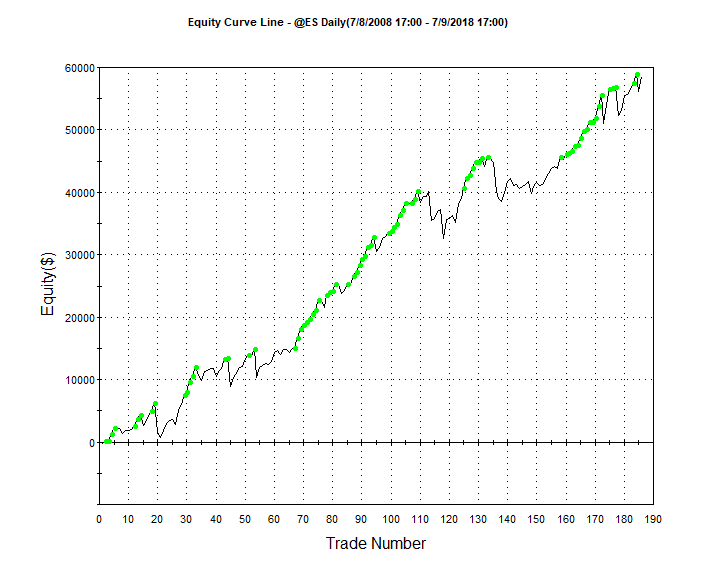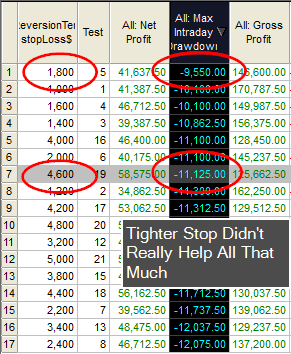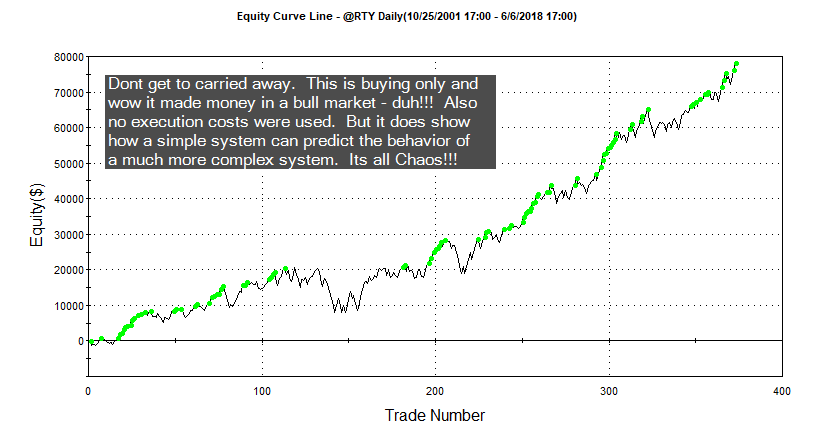# Anatomy Of Mean Reversion in EasyLanguage

Look at this equity curve:As long as you are in a bull market buying dips can be very consistent and profitable.  But you want to use some type of entry signal and trade management other than just buying a dip and selling a rally.  Here is the anatomy of a mean reversion trading algorithm that might introduce some code that you aren’t familiar.  Scroll through the code and I will  summarize below.

``````inputs: mavlen(200),rsiLen(2),rsiBuyVal(20),rsiSellVal(80),holdPeriod(5),stopLoss\$(4500);
vars: iCnt(0),dontCatchFallingKnife(false),meanRevBuy(false),meanRevSell(false),consecUpClose(2),consecDnClose(2);

Condition1 = c > average(c,mavLen);

Condition2 = rsi(c,rsiLen) < rsiBuyVal;
Condition3 = rsi(c,rsiLen) > rsiSellVal;

Value1 = 0;
Value2 = 0;

For iCnt = 0 to consecUpClose - 1
Begin
value1 = value1 + iff(c[iCnt] > c[iCnt+1],1,0);
end;

For iCnt = 0 to consecDnClose - 1
Begin
Value2 = value2 + iff(c[iCnt] < c[iCnt+1],1,0);
end;

dontCatchFallingKnife = absValue(C - c) < avgTrueRange(10)*2.0;

meanRevBuy = condition1 and condition2 and dontCatchFallingKnife;
meanRevSell =  not(condition1) and condition3 and dontCatchFallingKnife;

If meanRevBuy then buy this bar on close;
If marketPosition = 1 and condition1 and value1 >= consecUpClose then sell("ConsecUpCls") this bar on close;

If meanRevSell then sellShort this bar on close;
If marketPosition = -1 and not(condition1) and value2 >= consecDnClose then buyToCover this bar close;

setStopLoss(stopLoss\$);

If barsSinceEntry = holdPeriod then
Begin
if marketPosition = 1 and not(meanRevBuy) then sell this bar on close;
if marketPosition =-1 and not(meanRevSell) then buytocover this bar on close;
end;``````
Mean Reversion System

I am using a very short term RSI indicator, a la Connors, to initiate long trades.  Basically when the 2 period RSI dips below 30 and the close is above the 200-day moving average I will buy only if I am not buying “a falling knife.”  In February several Mean Reversion algos kept buying as the market fell and eventually got stopped out with large losses.  Had they held on they probably would have been OK.  Here I don’t buy if the absolute price difference between today’s close and yesterday’s is greater than 2 X the ten day average true range.  Stay away from too much “VOL.”

Once a trade is put on I use the following logic to keep track of consecutive closing relationships:

``````For iCnt = 0 to consecUpClose - 1
Begin
value1 = value1 + iff(c[iCnt] > c[iCnt+1],1,0);
end;``````
Using the IFF function in EasyLanguage

Here I am using the IFF function to compare today’s close with the prior day’s.  iCnt is a loop counter that goes from 0 to 1. IFF checks the comparison and if it’s true it returns the first value after the comparison and if false it returns the last value.  Here if I have two consecutive up closes value1 accumulates to 2.  If I am long and I have two up closes I get out.  With this template you can easily change this by modifying the input:  consecUpClose.  Trade management also includes a protective stop and a time based exit.  If six days transpire without two up closes then the system gets out – if the market can’t muster two positive closes, then its probably not going to go anywhere.  The thing with mean reversion, more so with other types of systems, is the use or non use of a protective stop.  Wide stops are really best, because you are betting on the market to revert.  Look at the discrepancy of results using different stop levels on this system:Here an \$1,800 stop only cut the max draw down by \$1,575.  But it came at a cost of \$17K in profit.  Stops, in the case of Mean Reversion, are really used for the comfort of the trader.

This code has the major components necessary to create a complete trading system.  Play around with the code and see if you can come up with a better entry mechanism.

# Update To Original Pattern Smasher

## What will you learn : string manipulation, for-loops, optimization

Before proceeding I would suggest reading my original post on this subject.    If you believe the relationship of the last few bars of data can help determine future market direction, then this post will be in you wheel house.  Another added benefit is that you will also learn some cool EasyLanguage.

### Original post was limited to four day patterns!

This version is limitless (well not really, but pretty close).  Let’s stick with the original string pattern nomenclature (+ + – – : two up closes followed by two down closes.)  Let’s also stick with our binary pattern representation:

 Pattern # 2^3 2^2 2^1 1 3 0 0 1 1 4 0 1 0 0 5 0 1 0 1 6 0 1 1 1

Remember a 0 represents a down close and a 1 represents an up close.  We will deviate from the original post by doing away with the array and stick with only strings (which are really just arrays of characters.)  This way we won’t have to worry about array manipulation.

#### How to create a dynamic length string pattern

This was the difficult part of the programming.  I wanted to be able to optimize 3, 4 and 5 day patterns and I wanted to control this with using just inputs.  I discovered that pattern three is different in a three day pattern than it is in a four day pattern: in a three day pattern it is 011 or – + + and in a four day pattern it is 0011 or – – + +.  Since I am counting 0’s as down closes, pattern #3 depends on the ultimate size of the pattern string.  No worries I will have eventually have another version where I utilize a different value for down closes and we can then have holes in our string patterns.  But I digress – so to differentiate the patterns based on the pattern length I included a maxPatternLen input.  So if maxPatternLen is three and we are trying to match pattern #3 then we will be looking for 011 and not 0011.  That was an easy fix.  But then I wanted to build a string pattern based on this input and the pattern number dynamically.  Here is some psuedo code on how I figured it out.

``````
{Psuedo code to translate pattern number into binary number}
patternNumber = 3
maxPatternLen = 3

numBits = 0    						// stick with binary representation
testValue = 0						// temporary test value
numBits = maxPatternLen-1  			// how many bits will it take to get to the
// center of - or numBits to represent max
// number of patterns or 2^numBits
currentBit =numBits					// start wit current bit as total numBits

value1 = patternOptTest				// value1 represents current pattern number
testString = ""  					// build test string from ground up

for icnt = numBits downto 0			//building string from left to right
begin       						// notice keyword downto
if power(2,currentBit) > value1 then  // must use power function in EL
begin							// if the very far left bit value >
testString = testString + "-"	  // patten number then plug in a "-"
end
else
begin							// else plug in a "+" and deccrement by
testString = testString + "+"	 // that bits value - if its the 3rd bit
value1 = value1 - power(2,currentBit)// then decrement by 8
end;
currentBit = currentBit - 1		// move onto the next bit to the right
end;``````
Pseudocode for Binary Representation of Pattern #

Now if you want to optimize then you must make sure your pattern number search space or range can be contained within maxPatternLen.  For example, if you want to test all the different combinations of a four day pattern, then your maxPatternLen would naturally be four and you would optimize the pattern number from 0 to 15.  Don’t use 1-16 as I use zero as the base.  A five day pattern would include the search space from 0 – 31.  The rest of the code was basically hacked from my original post.   Here is the rest of the code to do optimizations on different length pattern strings.  Notice how I use strings, for-loops and comparisons.

``````input: buyPattern("+++-"),sellPattern("---+"),patternOptimize(True),patternOptTest(7),maxPatternLen(3),patternOptBuySell(1),
stopLoss\$(2000),profitTarg\$(2000),holdDays(5);
vars: buyPatternString(""),sellPatternString(""),buyPatternMatch(""),sellPatternMatch(""),numBits(0),testValue(0),currentBit(0),
remainder(0),value(0),icnt(0),testString(""),numCharsInBuyPattern(0),numCharsInSellPattern(0);
vars:okToBuy(false),okToSell(false);

buyPatternMatch = buyPattern;
sellPatternMatch = sellPattern;
numCharsInBuyPattern = strLen(buyPatternMatch);
numCharsInSellPattern = strLen(sellPatternMatch);

If patternOptimize then
begin
numBits = 0;
testValue = 0;
value = maxPatternLen;
numBits = maxPatternLen-1;
currentBit =numBits;
remainder = patternOptTest;
testString = "";
for icnt = numBits downto 0
begin
if power(2,currentBit) > value1 then
begin
testString = testString + "-";
end
else
begin
testString = testString + "+";
remainder = remainder - power(2,currentBit);
end;
currentBit = currentBit - 1;
end;
numCharsInBuyPattern = maxPatternLen;
numCharsInSellPattern = maxPatternLen;
if patternOptBuySell = 1 then
Begin
buyPatternMatch = testString;
sellPatternMatch = "0";
end;
If patternOptBuySell = 2 then
Begin
buyPatternMatch = "0";
sellPatternMatch = testString;
end;
end;

buyPatternString = "";
sellPatternString = "";

For icnt = numCharsInBuyPattern-1 downto 0
Begin
If close[icnt] >= close[icnt+1] then buyPatternString = buyPatternString + "+";
If close[icnt] < close[icnt+1] then buyPatternString = buyPatternString + "-";
end;
For icnt = numCharsInSellPattern-1 downto 0
Begin
If close[icnt] >= close[icnt+1] then sellPatternString = sellPatternString + "+";
If close[icnt] < close[icnt+1] then sellPatternString = sellPatternString + "-";
end;

okToBuy = false;
okToSell = false;

if buyPatternMatch <> "" then
If buyPatternString = buyPatternMatch then okToBuy = true;
If buyPatternMatch = "" then
okToBuy = true;
If sellPattern <> "" then
If sellPatternString = sellPatternMatch then okToSell = true;
If sellPatternMatch = "" then
okToSell = true;

If okToBuy then buy next bar at open;
If okToSell then sellshort next bar at open;

If marketPosition = 1 and barsSinceEntry > holdDays then sell next bar at open;
If marketPosition = -1 and barsSinceEntry > holdDays then buytocover next bar at open;

setStopLoss(stopLoss\$);
setProfitTarget(profitTarg\$);

If lastBarOnChart then print(d," ",buyPatternMatch);``````
Final Version of New Pattern Smasher

Also see how I incorporate a profit target and protective stop.  I use the built in BarsSinceEntry function to count the number of days I am in a trade so I can utilize a time based exit.  Here is an interesting equity curve I developed using a two day pattern ( – –) to go long.Register on the website and I will email you an ELD of the improved Pattern Smasher.  Or just shoot me an email.